## Holyhedron!

A Holyhedron is a polyhedron in which every face has a "hole". Until recently it was unknown whether a finite holyhedron existed. Jade Vinson was the first to discover one, with 78,585,627 faces.

### A \$20 Holyhedron

Here is a holyhedron with 492 faces. John Conway has offered a prize of \$10000 divided by the number of faces, so this one should be worth approximately \$20.3252.

This holyhedron consists of nine layers. Each image in the first row shows a single one of these layers, while the corresponding image in the second row shows all layers up to and including that one. The final image in the lower right corner is the full holyhedron. Click on any of the small images to view a larger version of it.

12 faces 12*6 = 72 faces 20*4 = 80 faces 30*4 = 120 faces 12*6 = 72 faces 20*4 = 80 faces 4*4 = 16 faces 6*4 = 24 faces 4*4 = 16 faces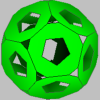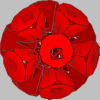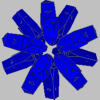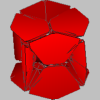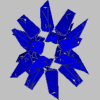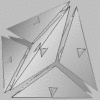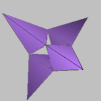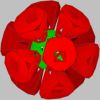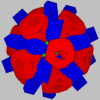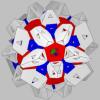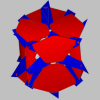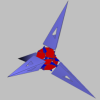Here is a cutaway view of the entire holyhedron (click on it for a larger image).The excellent program JavaView was used to model the holyhedron and to render these images.

The following graph shows the "piercing relation" among the layers; it is a schematic of which parts pierce which other parts of the holyhedron, and may be helpful in understanding what is going on in the pictures above. The nine nodes in the graph represent the nine layers of the holyhedron, colored the same as in the above images. The label on each graph edge is the number of piercings that the "from" layer makes into the "to" layer. The two graph edges leading nowhere from the High Spikes nodes represent the eight vertices on the convex hull of the holyhedron, which don't pierce anything.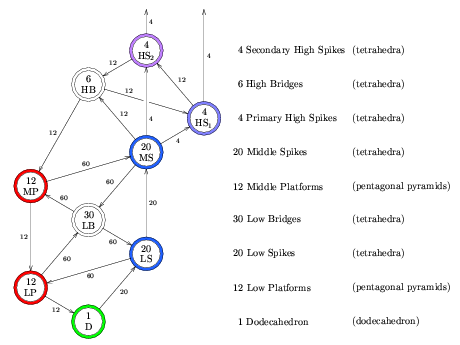Postscript version (suitable for printing)

Here are some vrml models of it. The "exploding" ones may or may not work in any particular vrml viewer; sorry, that's life.
Here are some other references to holyhedra on the web:

 Last Modified: Fri May 23 23:15:29 PDT 2003 Don Hatch hatch@plunk.org